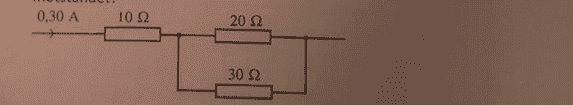# Calculate electric current in a resistor

• nowayn

## Homework Statementcalculate the electric current in the 20 ohm resistor

## The Attempt at a Solution

I have no idea where to start, but i thought maybe you should calculate the voltage first and then the voltage over the 20 ohm resistor.

## Homework Statementcalculate the electric current in the 20 ohm resistor

## The Attempt at a Solution

I have no idea where to start, but i thought maybe you should calculate the voltage first and then the voltage over the 20 ohm resistor.
You know the current through the parallel pair of resistors. How do you get from there to the current through the 20ohm resistor. You don't need voltage.

You know the current through the parallel pair of resistors.
wait so is the current there also 0,3A?

wait so is the current there also 0,3A?
Do you understand the concepts of series elements and parallel elements? If you are not clear on that then you need to GET clear on it before trying ANY problems of this sort, else you will be perpetually confused.

Investigate the topic: current divider.

The current divider and the voltage divider are two concepts that you will find to be very handy to know about.

You don't know where to start ? Start of by calculating the parallel resistance between 20ohm and 30ohm resistor, then maybe something will click off in your mind.

1/20=0,05
1/30=0,0333
0,08333/0,05=0,6
resistor with 20ohms will get 60% of the current
0,3*0,6=0,18